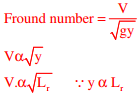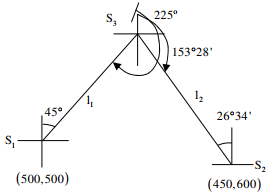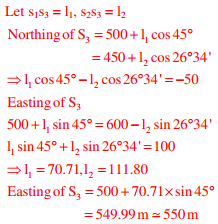# CE GATE 2015 - Online Test

Q1. SO2 and CO adversely affect
Explaination / Solution:

Carbon monoxide effects the bloods carrying capacity

Q2. Choose the statement where underlined word is used correctly
Explaination / Solution:

insured-the person, group, or organization whose life or property is covered by an insurance policy. ensured- to secure or guarantee

Q3. The relationship between the length scale ratio (Lr) and the velocity scale ratio (Vr) in hydraulic models, in which Froude dynamic similarity is maintained, is
Explaination / Solution:Q4.  The following statements are made related to the lengths of turning lanes at signalised intersections
(i) 1.5 times the average number of vehicles (by vehicle type) that would store in turning lane per cycle during the peak hour.
(ii) 2 times the average number of vehicles (by vehicle type) that would store in turning lane per cycle during the peak hour.
(iii) Average number of vehicles (by vehicle type) that would store in the adjacent through lane per cycle during the peak hour.
(iv) Average number of vehicles (by vehicle type) that would store in all lanes per cycle during the peak hour.
As per the IRC recommendations, the correct choice for design length of storage lanes is
Explaination / Solution:
No Explaination.

Q5. A hydraulic jump takes place in a frictionless rectangular channel. The pre-jump depth is yp. The alternate and sequent depths corresponding to yp are ya and ys respectively. The correct relationship among yp, ya and ys is
Explaination / Solution:
No Explaination.

Q6. The bearings of two inaccessible stations, S1 (Easting 500 m, Northing 500 m) and S2 (Easting 600 m, Northing 450 m) from a station S3 were observed as 225° and 153° 26′ respectively. The independent Easting (in m) of station S3 is:
Explaination / Solution:Q7. In friction circle method of slope stability analysis, if r defines the radius of the slip circle, the radius of friction circle is
Explaination / Solution:
No Explaination.

Q8. The combined correction due to curvature and refraction (in m) for distance of 1 km on the surface of Earth is
Explaination / Solution:

C = 0.0673d2 = 0.0673×1

Q9. In the water content of a fully saturated soil mass is 100% the void ratio of the sample is
Explaination / Solution:
No Explaination.

Q10. A 6 m high retaining wall having a smooth vertical back face retains a layered horizontal backfill. Top 3 m thick layer of the backfill is sand having an angle of internal friction, φ = 30° while the bottom layer is 3 m thick clay with cohesion, c = 20 kPa. Assume unit weight for both sand and clay as 18 kN/m3. The totalactive earth pressure per unit length of the wall (in kN/m) is:
Explaination / Solution:
No Explaination.